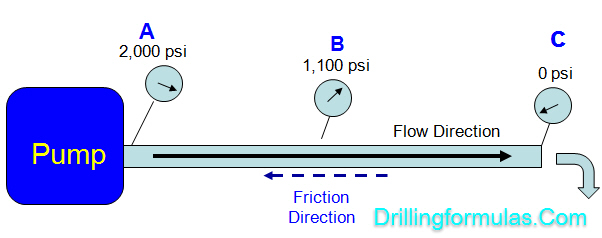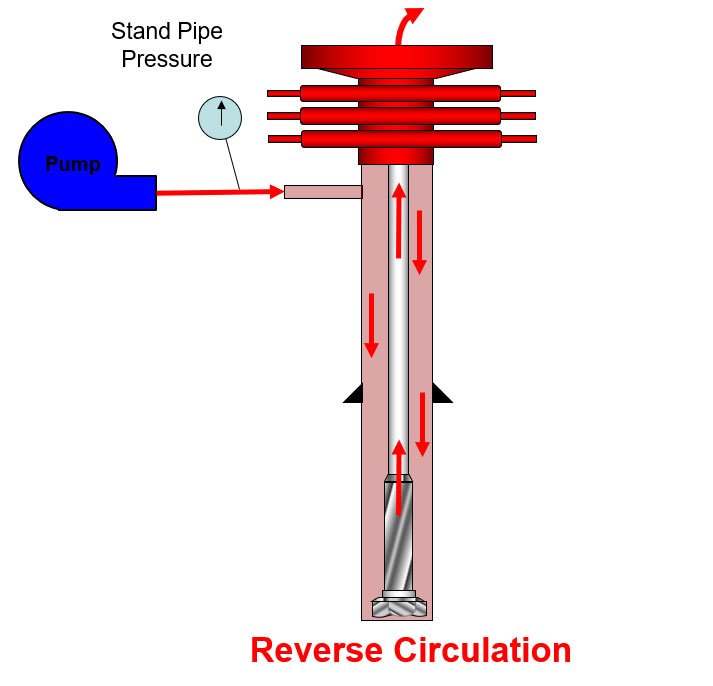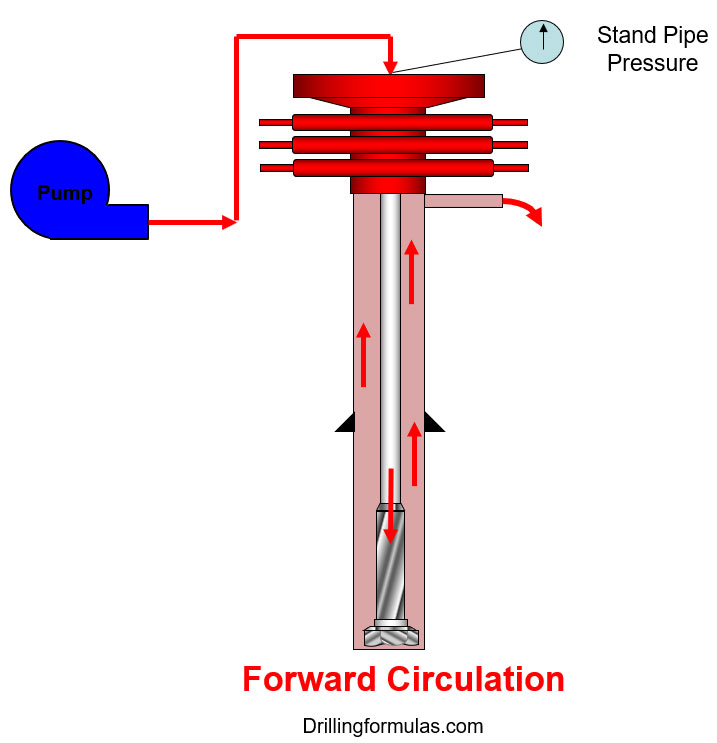## Understand Pressure Loss (Frictional Pressure) in Drilling System

Frictional pressure is pressure loss acting in the opposite direction of fluid flow and today we will look into each component in pressure therefore you will get clearer picture about the frictional pressure in drilling system.

Let take a look at the simple diagram below.A mud pump creates power to move drilling fluid from point A to C and the frictional pressure or pressure loss is the amount of pressure required to transfer fluid.

Pump pressure is 2,000 psi at the starting point (“A”) and at the end point (“C”), pressure is 0 psi. This tells you that you need 2,000 psi to overcome the frictional pressure in order to move the fluid from point “A” to point “C”.

“Differential pressure between two points in the system is pressure loss while fluid is moved from one to another point.”

## Effect of Frictional Pressure on ECD while Reverse circulationA reverse circulation is another way to circulate by circulating into annulus up to a bit and drill string. The fluid outlet is on surface. For drilling operation, we most of the time use forward circulation; however, in completion operation, the reverse circulation is utilized more often. For more understanding, we would like to show an image below (Figure 1) which demonstrates a flow path of the reverse circulation.Figure 1 – Reverse Circulation

## Effect of Frictional Pressure on ECD while Forward CirculationIn this article, we will describe the effect of friction pressure on bottom hole pressure and equivalent circulating density while performing forward circulation.

### What is forward circulation?

It is the typical circulating path which is from a mud pump into drill pipe. Mud is pumped down into drill string / BHA and come out of a bit.  Then, the mud is flown up the annulus and return back to surface as you can see in the diagram below (Figure 1).Figure 1 – Forward Circulation Path